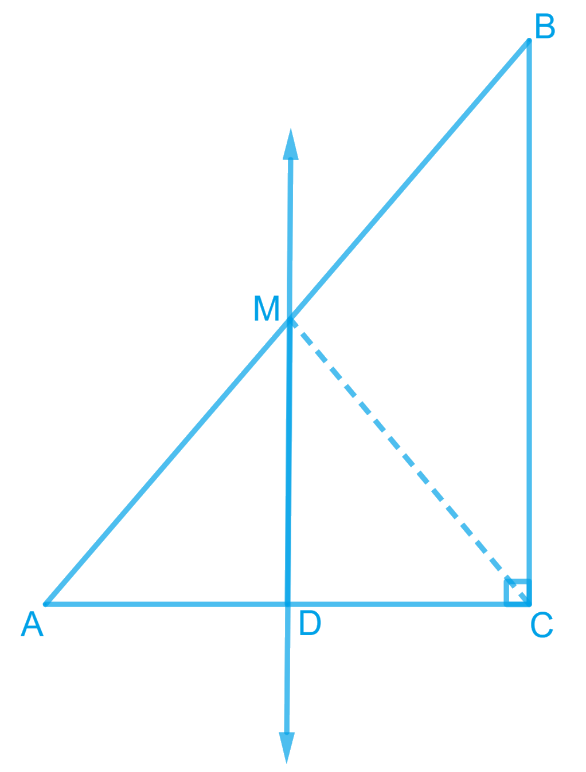In the verge of coronavirus pandemic, we are providing FREE access to our entire Online Curriculum to ensure Learning Doesn't STOP!

# Ex.8.2 Q7 Quadrilaterals Solution - NCERT Maths Class 9

Go back to  'Ex.8.2'

## Question

$$ABC$$ is a triangle right angled at $$C$$. $$A$$ line through the mid-point $$M$$ of hypotenuse $$AB$$ and parallel to $$BC$$ intersects $$AC$$ at $$D$$. Show that

(i) $$D$$ is the mid-point of $$AC$$

(ii) $$\rm{MD} \perp \rm{AC}$$

(iii)  \begin{align} \rm{CM}=\rm{MA}=\frac{1}{2} \rm{AB} \end{align}

Video Solution
Ex 8.2 | Question 7

## Text Solution

What is known?

$$ABC$$ is a triangle right angled at $$C$$. A line through the mid-point M of hypotenuse $$AB$$ and parallel to $$BC$$ intersects $$AC$$ at $$D.$$

What is unknown?

How we can show that

(i) $$D$$ is the mid-point of $$AC$$

(ii) $${MD }\!\!~\!\!\bot {AC}$$

(iii) $${CM}={MA}=\frac{1}{2}{ AB}$$

Reasoning:

By converse of mid-point theorem, we know that a line drawn through the mid-point of any side of a triangle and parallel to another side, bisects the third side. To show $$MD$$ perpendicular to $$AC$$ we know that sum of interior angles is $$180$$. Also to get $$CM=MA=$$half of AB we can show two triangles congruent

Steps:(i) In $$\Delta {ABC,}$$

It is given that $$M$$ is the mid-point of $$AB$$ and $$MD$$ $$\parallel$$ $$BC$$.

Therefore, $$D$$ is the mid-point of $$AC$$. (Converse of mid-point theorem)

(ii) As $$DM$$ $$\parallel$$ $$CB$$ and $$AC$$ is a transversal line for them, therefore,

\begin{align} &\angle {MDC}+\angle {DCB}=180^{\circ}\\&({Co} \text { -interior angles })\\ &\angle {MDC}+90^{\circ}=180^{\circ}\\ &\angle {MDC}=90^{\circ}\\ &\therefore {MD} \perp {AC}\end{align}

(iii) Join $$MC$$.

In $$\Delta {AMD}$$ and $$\Delta {CMD}$$

$$AD = CD$$ (D is the mid-point of side $$AC$$)

\begin{align} \angle ADM= \angle CDM \; (Each\; 90^0 ) \end{align}

$$DM = DM$$ (Common)

\begin{align} \therefore \Delta {AMD}\cong \Delta {CMD}\end{align} (By SAS congruence rule)

Therefore, $$AM = CM$$ (By CPCT)

However, \begin{align} AM = \frac{1}{2} AB \end{align} (M is the mid-point of AB)

Therefore, it can be said that

\begin{align} CM= AM = \frac{1}{2} AB \end{align}

Video Solution